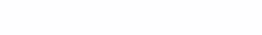# Expanding the boundaries or about the problem of testing the hypothesis about the normality of a multivariate distribution

A short story about the MVN package

A moment of theory

Let’s say we have some joint distribution of n variables – and we need to check if it is normal. One small fact simply prevents us from solving this problem – from the normality of the multivariate distribution, the normality of the distribution of each variable separately follows, but in the opposite direction it works only if the components of the distribution are independent, which in practice is almost never fulfilled. Therefore, you have to invent something.

The scheme for testing the statistical hypothesis about the normality of the multivariate distribution is identical to the corresponding one for the one-dimensional case, only it uses different tests.

The Mardia test (original work: KV Mardia. Measures of multivariate skewness and kurtosis with applications. Biometrika, 57 (3): 519-530, 1970) is based on calculating the kurtosis and skewness of the multivariate distribution by the formulas

Moreover, m is the Malanhobis distance between the i-th and j-th observations

In this interpretation, the calculated asymmetry value multiplied by n / 6 is distributed according to the Chi-square law with p (p + 1) (p + 2) / 6 degrees of freedom, and the kurtosis value is distributed according to the normal distribution law with the mean p (p + 2) and deviation 8p (p + 2) / n

Henze-Zirkler test (base work: N. Henze and B. Zirkler. A class of invariant consistent tests for multivariate normality. Communications in Statistics – Theory and Methods, 19 (10): 3595–3617, 1990.) is based on the following formula calculating the statistical criterion:

The values ​​of the criterion are distributed according to the lognormal law with the parameters

Royston’s test is based on the idea of ​​the Shapiro-Wilks test. The value of the statistical criterion is calculated by the formula

Its value is distributed according to the Chi-square law with the number of degrees of freedom equal to e. The chain of calculations is as follows:Wj is the value of the Shapiro-Wilk statistic for the j-th variable, r is the correlation coefficient

The Dornik-Hansen test (original work: Doornik, JA, and H. Hansen. 2008. An omnibus test for univariate and multivariate normality. Oxford Bulletin of Economics and Statistics 70: 927–939.) Is based on transforming multivariate observations and calculating the kurtosis and asymmetry for a one-dimensional variable.

The transformation is carried out according to the formulaThe first matrix of this product is the centered matrix of the initial data X The second matrix is ​​a diagonal matrix in which the elements are equal to S-1/2 for a separate variable The third matrix is ​​the matrix of eigenvectors of the correlation matrix C The fourth matrix is ​​the diagonal matrix of eigenvalues ​​of matrix C

Next, the kurtosis and skewness are calculated for each variable in the new matrix.

The asymmetry values ​​(bone) and kurtosis (b2) are not distributed according to the normal law. To transform them, the following transformations are applied:

The obtained values ​​of zone and z2 are combined into vectors Zone and Z2, and the calculated value of the statistic is distributed according to the Chi-square law with the number of degrees of freedom equal to 2k

The E-statistic test (Shekeli-Rizzo test, basic work: GJ Szekely, ML Rizzo. A new test for multivariate normality / Journal of Multivariate Analysis 93 (2005) 58–80) implies the calculation of test statistics using the Taylor series expansion:

y is the centered observation matrix obtained by column-wise transformations as

Methodology

For example, let’s select the “Crime” database from the plm package, and take three variables from there:

prbpris – probability of imprisonment

avgsen – average term of imprisonment, days

pctymle – share in the population of men aged 15-24 years

From these three variables, we will collect two databases – with two and three variables:

``````library(MVN)
library(tidyverse)
library(plm)
data("Crime")
glimpse(Crime)
ggplot(Crime, aes(x=Crime\$avgsen)) + geom_density()
# Crime\$prbpris - точно, avgsen - 70/30, pctymle - 50/50
Data_1 <- Crime[,c(6,7)]
Data_2 <- Crime[,c(6,7,24)]``````

Calculations and description

The basic calculation function is the mvn function with the following parameters:

data – Database (in the form of a matrix or dataframe)

subset – Factorial grouping variable

mvnTest – Specifies the statistical test to be checked

desc – Boolean variable. If it is true, descriptive statistics are output.

univariateTest – Defines a statistical test that checks the normality of individual variables

univariatePlot – Determines the kind of univariate normality plot to display

multivariatePlot – Determines the appearance of the error plot

multivariateOutlierMethod – Selects the method for determining outliers

Let’s check our data for normality using the classic Mardia testThe result is the same – NO in the “Result” and “Normality” columns tell us that it is impossible to accept the hypothesis about the normal multivariate distribution of both the entire set of variables and the normal distribution of each variable separately.

You can look at the QQ chart with your eyes

``mvn(data = Data_1, mvnTest = "mardia", multivariatePlot = "qq")``

And also on a two-dimensional distribution graph

``mvn(data = Data_1, mvnTest = "mardia", multivariatePlot = "qq")``

And on a 2D contour plot

``mvn(data = Data_1, mvnTest = "energy", multivariatePlot = "contour")``
``mvn(data = Data_1, mvnTest = "mardia", univariatePlot = "qqplot")``